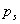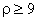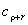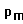Generalized Polygonal Wankel Engines

Emil Molnár and Jenő Szirmai

Department of Geometry, Institute of Mathematics

Budapest University of Technology and Economics

The trigonal Wankel engine is kinematically based on the motion where a circleof radius 3d, as the moving pool curve, rolles on the circleof radius 2d, as the standing pole curve in the interior. Then the regular trigonal rotor with outcircle of radius r>3d, fixed concentrically the moving pole circle, describes its orbit curve. This orbit curveis crutial in forming the engine space.

Answering a question of  István Revuczky  we prove and animate by computer thatis a convex curve iff. The parallel curvewith distance r will be the solution to the engine space if the triangle rotor toucheswith small roller circles of radius r) centred in the vertices of the triangle.

All these concepts will be generalized -- with animation -- to a k-gonal rotor(, natural numbers) in a natural way.

The moving and the standing pole curves

Animation: [k=3-t],      The moving pole curverolles ont he standing pole curve. The moving pole curve is a circle of the critical radius kd, The standing pole curve is of radius       (k-1)d  ( here k=3, d=1, r=6).

Animation: [k=4-t],      The moving pole curverolles ont he standing pole curve. The moving pole curve is a circle of the critical radius kd, The standing pole curve is of radius       (k-1)d  ( here k=4, d=1, r=7).

Konvex and non-konvex motor spaces

Anaimations for the parameters: [k=3],  [k=4],  [k=5],  [k=8].

You can download the fileElliptor zipwith the animations to a directory on your hard drive. The animations have been created with Maple 7.